CHIRAG GOYAL — Published On May 28, 2021 and Last Modified On June 24th, 2022

This article was published as a part of the Data Science Blogathon

## Introduction

Logistic Regression, a statistical model is a very popular and easy-to-understand algorithm that is mainly used to find out the probability of an outcome.

Therefore it becomes necessary for every aspiring Data Scientist and Machine Learning Engineer to have a good knowledge of Logistic Regression.

In this article, we will discuss the most important questions on Logistic Regression which is helpful to get you a clear understanding of the techniques, and also for Data Science Interviews, which covers its very fundamental level to complex concepts.

## 1. What do you mean by the Logistic Regression?

It’s a classification algorithm that is used where the target variable is of categorical nature. The main objective behind Logistic Regression is to determine the relationship between features and the probability of a particular outcome.

For Example, when we need to predict whether a student passes or fails in an exam given the number of hours spent studying as a feature, the target variable comprises two values i.e. pass and fail.

Therefore, we can solve classification problem statements which is a supervised machine learning technique using Logistic Regression.

## 2. What are the different types of Logistic Regression?

Three different types of Logistic Regression are as follows:

1. Binary Logistic Regression: In this, the target variable has only two 2 possible outcomes.

For Example, 0 and 1, or pass and fail or true and false.

2. Multinomial Logistic Regression: In this, the target variable can have three or more possible values without any order.

For Example, Predicting preference of food i.e. Veg, Non-Veg, Vegan.

3. Ordinal Logistic Regression: In this, the target variable can have three or more values with ordering.

For Example, Movie rating from 1 to 5.

## 3. Explain the intuition behind Logistic Regression in detail.

Given:

By using the training dataset, we can find the dependent(x) and independent variables(y), so if we can determine the parameters w (Normal) and b (y-intercept), then we can easily find a decision boundary that can almost separate both the classes in a linear fashion.

Objective:

In order to train a Logistic Regression model, we just need w and b to find a line(in 2D), plane(3D), or hyperplane(in more than 3-D dimension) that can separate both the classes point as perfect as possible so that when it encounters with any new unseen data point, it can easily classify, from which class the unseen data point belongs to.

For Example, Let us consider we have only two features as x1 and x2.

Let’s take any of the +ve class points (figure below) and find the shortest distance from that point to the plane. Here, the shortest distance is computed using:

di = wT*xi / ||w||

If weight vector is a unit vector i.e, ||w||=1. Then,

di = wT*xi

Since w and xi are on the same side of the decision boundary therefore distance will be +ve. Now for a negative point, we have to compute dj = wT*xj. For point xj, distance will be -ve since this point is the opposite side of w.

Thus we can conclude, points that are in the same direction of w are considered as +ve points and the points which are in the opposite direction of w are considered as -ve points.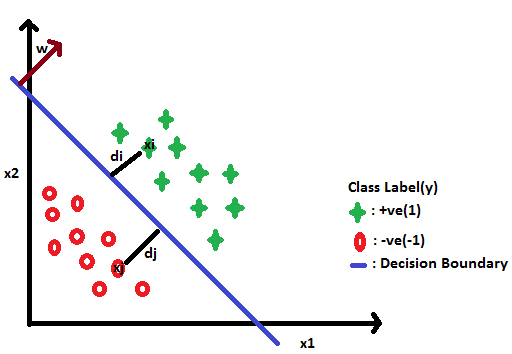Now, we can easily classify the unseen data points as -ve and +ve points. If the value of wT*xi>0, then y =+1 and if value of wT*xi < 0 then y = -1.

• If yi = +1 and wT*xi > 0, then the classifier classifies it as+ve points. This implies if yi*wT*xi > 0, then it is a correctly classified point because multiplying two +ve numbers will always be greater than 0.
• If yi = -1 and wT*xi < 0, then the classifier classifies it as -ve point. This implies if yi * wT*xi > 0 then it is a correctly classified point because multiplying two -ve numbers will always be greater than zero. So, for both +ve and -ve points the value of yi* wT*xi is greater than 0. Therefore, the model classifies the points xi correctly.
• If yi = +1 and wT*xi < 0, i.e, yi is +ve point but the classifier says that it is -ve then we will get -ve value. This means that point is classified as -ve but the actual class label is +ve, then it is a miss-classified point.
• If yi = -1 and wT*xi > 0, this means actual class label is -ve but classified as +ve, then it is miss-classified point( yi*wT*xi < 0).

Now, by observing all the cases above now our objective is that our classifier minimizes the miss-classification error, i.e, we want the values of yi*wT*xi to be greater than 0.

In our problem, xi and yi are fixed because these are coming from the dataset.

As we change the values of the parameters w, and b the sum will change and we want to find that w and b that maximize the sum given below. To calculate the parameters w and b, we can use the Gradient Descent optimizer. Therefore, the optimization function for logistic regression is: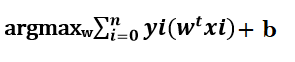## 4. What are the odds?

Odds are defined as the ratio of the probability of an event occurring to the probability of the event not occurring.

For Example, let’s assume that the probability of winning a game is 0.02. Then, the probability of not winning is 1- 0.02 = 0.98.

• The odds of winning the game= (Probability of winning)/(probability of not winning)
• The odds of winning the game= 0.02/0.98
• The odds of winning the game are 1 to 49, and the odds of not winning the game are 49 to 1.

## 5. What factors can attribute to the popularity of Logistic Regression?

Logistic Regression is a popular algorithm as it converts the values of the log of odds which can range from -inf to +inf to a range between 0 and 1.

Since logistic functions output the probability of occurrence of an event, they can be applied to many real-life scenarios therefore these models are very popular.

## 6. Is the decision boundary Linear or Non-linear in the case of a Logistic Regression model?

The decision boundary is a line or a plane that separates the target variables into different classes that can be either linear or nonlinear. In the case of a Logistic Regression model, the decision boundary is a straight line.

Logistic Regression model formula = α+1X1+2X2+….+kXk. This clearly represents a straight line.

It is suitable in cases where a straight line is able to separate the different classes. However, in cases where a straight line does not suffice then nonlinear algorithms are used to achieve better results.

## 7. What is the Impact of Outliers on Logistic Regression?

The estimates of the Logistic Regression are sensitive to unusual observations such as outliers, high leverage, and influential observations. Therefore, to solve the problem of outliers, a sigmoid function is used in Logistic Regression.

## 8. What is the difference between the outputs of the Logistic model and the Logistic function?

The Logistic model outputs the logits, i.e. log-odds; whereas the Logistic function outputs the probabilities.

Logistic model = α+1X1+2X2+….+kXk. Therefore, the output of the Logistic model will be logits.

Logistic function = f(z) = 1/(1+e-(α+1X1+2X2+….+kXk)). Therefore, the output of the Logistic function will be the probabilities.

## 9. How do we handle categorical variables in Logistic Regression?

The inputs given to a Logistic Regression model need to be numeric. The algorithm cannot handle categorical variables directly. So, we need to convert the categorical data into a numerical format that is suitable for the algorithm to process.

Each level of the categorical variable will be assigned a unique numeric value also known as a dummy variable. These dummy variables are handled by the Logistic Regression model in the same manner as any other numeric value.

## 10. Which algorithm is better in the case of outliers present in the dataset i.e., Logistic Regression or SVM?

SVM (Support Vector Machines) handles the outliers in a better manner than the Logistic Regression.

Logistic Regression: Logistic Regression will identify a linear boundary if it exists to accommodate the outliers. To accommodate the outliers, it will shift the linear boundary.

SVM: SVM is insensitive to individual samples. So, to accommodate an outlier there will not be a major shift in the linear boundary. SVM comes with inbuilt complexity controls, which take care of overfitting, which is not true in the case of Logistic Regression.

## 11. What are the assumptions made in Logistic Regression?

Some of the assumptions of Logistic Regression are as follows:

1. It assumes that there is minimal or no multicollinearity among the independent variables i.e, predictors are not correlated.

2. There should be a linear relationship between the logit of the outcome and each predictor variable. The logit function is described as logit(p) = log(p/(1-p)), where p is the probability of the target outcome.

3. Sometimes to predict properly, it usually requires a large sample size.

4. The Logistic Regression which has binary classification i.e, two classes assume that the target variable is binary, and ordered Logistic Regression requires the target variable to be ordered.

For example, Too Little, About Right, Too Much.

5. It assumes there is no dependency between the observations.

## 12. Can we solve the multiclass classification problems using Logistic Regression? If Yes then How?

Yes, in order to deal with multiclass classification using Logistic Regression, the most famous method is known as the one-vs-all approach. In this approach, a number of models are trained, which is equal to the number of classes. These models work in a specific way.

For Example, the first model classifies the datapoint depending on whether it belongs to class 1 or some other class(not class 1); the second model classifies the datapoint into class 2 or some other class(not class 2) and so-on for all other classes.

So, in this manner, each data point can be checked over all the classes.

## 13. How can we express the probability of a Logistic Regression model as conditional probability?

We define probability P(Discrete value of Target variable | X1, X2, X3…., Xk) as the probability of the target variable that takes up a discrete value (either 0 or 1 in the case of binary classification problems) when the values of independent variables are given.

For Example, the probability an employee will attain (target variable) given his attributes such as his age, salary, etc.

## 14. Discuss the space complexity of Logistic Regression.

During training: We need to store four things in memory: x, y, w, and b during training a Logistic Regression model.

• Storing b is just 1 step, i.e, O(1) operation since b is a constant.
• x and y are two matrices of dimension (n x d) and (n x 1) respectively. So, storing these two matrices takes O(nd + n) steps.
• Lastly, w is a vector of size-d. Storing it in memory takes O(d) steps.

Therefore, the space complexity of Logistic Regression while training is O(nd + n +d).

During Runtime or Testing: After training the model what we just need to keep in memory is w. We just need to perform wT*xi to classify the points.

Hence, the space complexity during runtime is in the order of d, i.e, O(d).

## 15. Discuss the Test or Runtime complexity of Logistic Regression.

At the end of the training, we test our model on unseen data and calculate the accuracy of our model. At that time knowing about runtime complexity is very important. After the training of Logistic Regression, we get the parameters w and b.

To classify any new point, we have to just perform the operation wT * xi. If wT*xi>0, the point is +ve, and if wT*xi < 0, the point is negative. As w is a vector of size d, performing the operation wT*xi takes O(d) steps as discussed earlier.

Therefore, the testing complexity of the Logistic Regression is O(d).

Hence, Logistic Regression is very good for low latency applications, i.e, for applications where the dimension of the data is small.

## 16. Why is Logistic Regression termed as Regression and not classification?

The major difference between Regression and classification problem statements is that the target variable in the Regression is numerical (or continuous) whereas in classification it is categorical (or discrete).

Logistic Regression is basically a supervised classification algorithm. However, the Logistic Regression builds a model just like linear regression in order to predict the probability that a given data point belongs to the category numbered as “1”.

For Example, Let’s have a binary classification problem, and ‘x’ be some feature and ‘y’ be the target outcome which can be either 0 or 1.

The probability that the target outcome is 1 given its input can be represented as: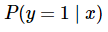If we predict the probability by using linear Regression, we can describe it as: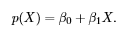where, p(x) = p(y=1|x)

Logistic regression models generate predicted probabilities as any number ranging from neg to pos infinity while the probability of an outcome can only lie between 0< P(x)<1.

However, to solve the problem of outliers, a sigmoid function is used in Logistic Regression. The Linear equation is put in the sigmoid function.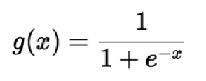## 17. Discuss the Train complexity of Logistic Regression.

In order to train a Logistic Regression model, we just need w and b to find a line(in 2-D), plane(in 3-D), or hyperplane(in more than 3-D dimension) that can separate both the classes point as perfect as possible so that when it encounters with any new point, it can easily classify, from which class the unseen data point belongs to.

The value of w and b should be such that it maximizes the sum yi*wT*xi > 0.

Now, let’s calculate its time complexity in terms of Big O notation:

• Performing the operation yi*wT*xi takes O(d) steps since w is a vector of size-d.
• Iterating the above step over n data points and finding the maximum sum takes n steps.Therefore, the overall time complexity of the Logistic Regression during training is n(O(d))=O(nd).

## 18. Why can’t we use Mean Square Error (MSE) as a cost function for Logistic Regression?

In Logistic Regression, we use the sigmoid function to perform a non-linear transformation to obtain the probabilities. If we square this nonlinear transformation, then it will lead to the problem of non-convexity with local minimums and by using gradient descent in such cases, it is not possible to find the global minimum. As a result, MSE is not suitable for Logistic Regression.

So, in the Logistic Regression algorithm, we used Cross-entropy or log loss as a cost function. The property of the cost function for Logistic Regression is that:

• The confident wrong predictions are penalized heavily
• The confident right predictions are rewarded less

By optimizing this cost function, convergence is achieved.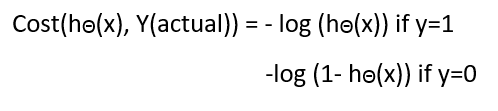## 19. Why can’t we use Linear Regression in place of Logistic Regression for Binary classification?

Linear Regressions cannot be used in the case of binary classification due to the following reasons:

1. Distribution of error terms: The distribution of data in the case of Linear and Logistic Regression is different. It assumes that error terms are normally distributed. But this assumption does not hold true in the case of binary classification.

2. Model output: In Linear Regression, the output is continuous(or numeric) while in the case of binary classification, an output of a continuous value does not make sense. For binary classification problems, Linear Regression may predict values that can go beyond the range between 0 and 1. In order to get the output in the form of probabilities, we can map these values to two different classes, then its range should be restricted to 0 and 1. As the Logistic Regression model can output probabilities with Logistic or sigmoid function, it is preferred over linear Regression.

3. The variance of Residual errors: Linear Regression assumes that the variance of random errors is constant. This assumption is also not held in the case of Logistic Regression.

## 20. What are the advantages of Logistic Regression?

The advantages of the logistic regression are as follows:

1. Logistic Regression is very easy to understand.

2. It requires less training.

3. It performs well for simple datasets as well as when the data set is linearly separable.

4. It doesn’t make any assumptions about the distributions of classes in feature space.

5. A Logistic Regression model is less likely to be over-fitted but it can overfit in high dimensional datasets. To avoid over-fitting these scenarios, One may consider regularization.

6. They are easier to implement, interpret, and very efficient to train.

## 21. What are the disadvantages of Logistic Regression?

The disadvantages of the logistic regression are as follows:

1. Sometimes a lot of Feature Engineering is required.

2. If the independent features are correlated with each other it may affect the performance of the classifier.

3. It is quite sensitive to noise and overfitting.

4. Logistic Regression should not be used if the number of observations is lesser than the number of features, otherwise, it may lead to overfitting.

5. By using Logistic Regression, non-linear problems can’t be solved because it has a linear decision surface. But in real-world scenarios, the linearly separable data is rarely found.

6. By using Logistic Regression, it is tough to obtain complex relationships. Some algorithms such as neural networks, which are more powerful, and compact can easily outperform Logistic Regression algorithms.

7. In Linear Regression, there is a linear relationship between independent and dependent variables but in Logistic Regression, independent variables are linearly related to the log odds (log(p/(1-p)).

## End Notes

I hope you enjoyed the questions and were able to test your knowledge about Logistic Regression.

If you liked this and want to know more, go visit my other articles on Data Science and Machine Learning by clicking on the Link

Something not mentioned or want to share your thoughts? Feel free to comment below And I’ll get back to you.

## Chirag Goyal

Currently, I am pursuing my Bachelor of Technology (B.Tech) in Computer Science and Engineering from the Indian Institute of Technology Jodhpur(IITJ). I am very enthusiastic about Machine learning, Deep Learning, and Artificial Intelligence.

The media shown in this article are not owned by Analytics Vidhya and is used at the Author’s discretion.###### CHIRAG GOYAL

I am currently pursuing my Bachelor of Technology (B.Tech) in Computer Science and Engineering from the Indian Institute of Technology Jodhpur(IITJ). I am very enthusiastic about Machine learning, Deep Learning, and Artificial Intelligence. Feel free to connect with me on Linkedin.